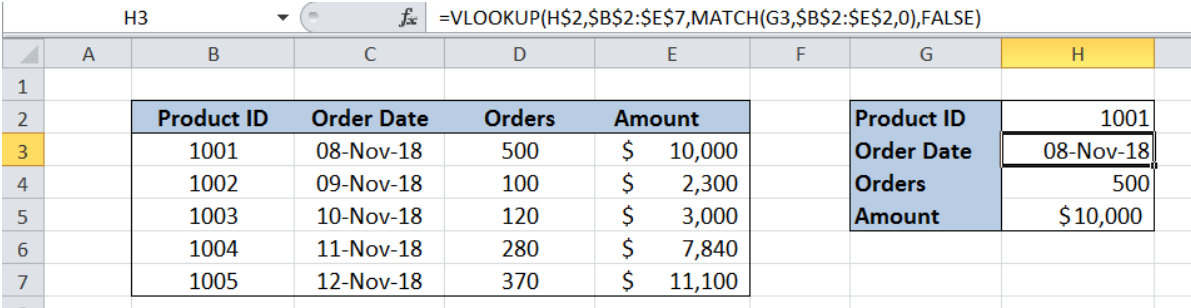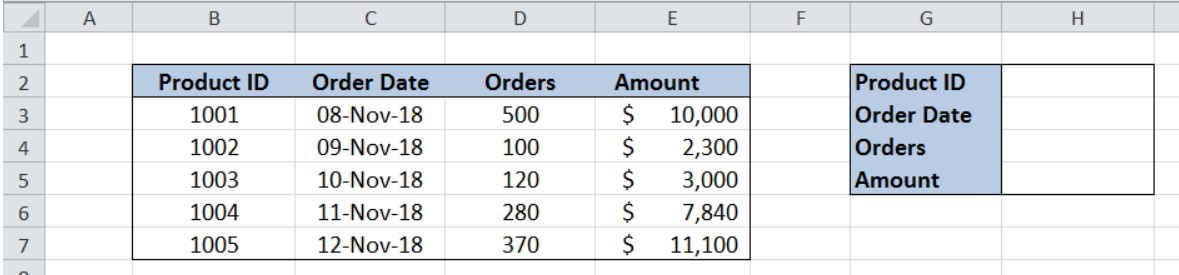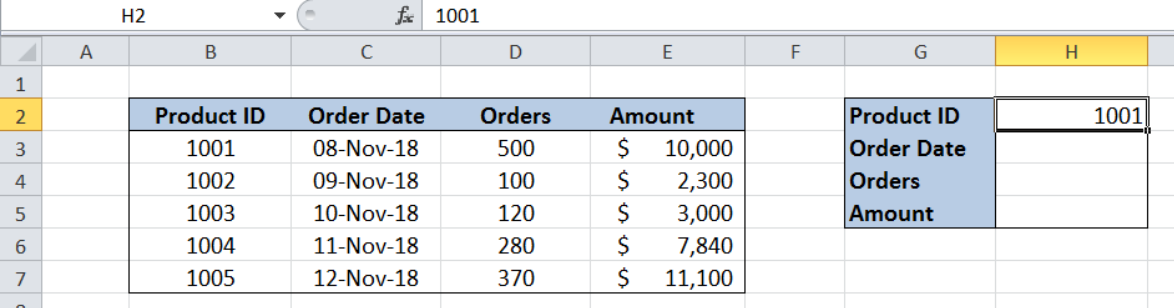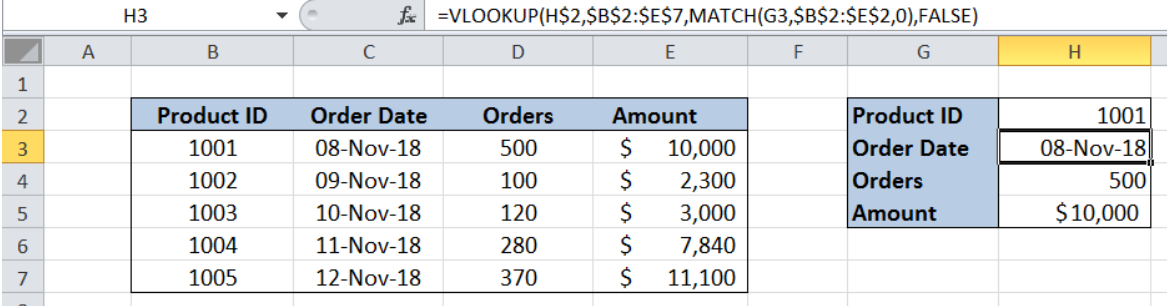Get instant live expert help with Excel or Google Sheets“My Excelchat expert helped me in less than 20 minutes, saving me what would have been 5 hours of work!”

#### Post your problem and you’ll get Expert help in seconds.

Your message must be at least 40 characters
Our professional Expert are available now. Your privacy is guaranteed.

# Two-way Lookup using VLOOKUP in a Table

While working with Excel, we normally have to work with more than one table.  Organizing and completing data in Excel can be done using the VLOOKUP and MATCH functions. This step by step tutorial will assist all levels of Excel users in retrieving data from one table to another by using a two-way lookup.Figure 1. Final result: Two-way lookup using VLOOKUP in a table

Final formula: `=``VLOOKUP(H\$2,\$B\$2:\$E\$7,MATCH(G3,\$B\$2:\$E\$2,0),FALSE)`

## Syntax of the VLOOKUP function

VLOOKUP is used when we want to look up and retrieve data from a given data set.

### Syntax

`=VLOOKUP(lookup_value, table_array, col_index_num, [range_lookup])`

The parameters of the VLOOKUP function are:

• lookup_value: the value we want to search and find in another table
• tale_array:    the range of cells in another table containing the data we want to retrieve
• col_index_num: the column number in the table_array corresponding to the information we want to retrieve, relative to the lookup_value
• [range_lookup]: optional; value is either TRUE or FALSE;
• if TRUE or omitted, VLOOKUP returns either an exact or approximate match
• if FALSE, VLOOKUP will only find an exact match

## Syntax of the MATCH function

The MATCH function returns the position of a value in a range.

### Syntax

`=MATCH(lookup_value, lookup_array, [match_type])`

The parameters of the MATCH function are:

• lookup_value – a value which we want to find in the lookup_array
• lookup_array – the range of cells containing the value we want to match
• [match_type] optional; the type of match; if omitted, the default value is 1; We use 0 to find an exact match

## Setting up the Data for Two-way Lookup

Below table has four columns:“Product ID” (column B), “Order Date (column C), “Orders” (column D) and “Amount” (column E).

In column H, we want to complete the fields Order Date, Orders and Amount per Product ID from the lookup table B2:E7.Figure 2. Sample data for the two-way lookup using VLOOKUP

## Two-way Lookup Using VLOOKUP

We will be using the VLOOKUP function to retrieve data from the table B2:E7.  We will combine the VLOOKUP function with the MATCH function to determine the column index number of each field with respect to the lookup value Product ID.

### Formula:

`=VLOOKUP(H\$2,\$B\$2:\$E\$7,MATCH(G3,\$B\$2:\$E\$2,0),FALSE)`

The lookup value is H2 or the Product ID.  The table array is the range B2:E7. The result of the MATCH formula MATCH(G3,\$B\$2:\$E\$2,0) returns the column number of the required field with respect to the Product ID in the table array.  Note that the cells are fixed using the dollar sign “\$” so that it will be easier to copy and paste the formula to other cells.

To perform the two-way lookup, we follow these steps:

Step 1. Enter Product ID “1001” in cell H2.Figure 3. Entering Product ID as the lookup value

Step 2. Select cell H3 and enter the formula: `=VLOOKUP(H\$2,\$B\$2:\$E\$7,MATCH(G3,\$B\$2:\$E\$2,0),FALSE)`

Step 3. Press ENTER

Step 4. Copy and paste the formula to the other cells H4 and H5 by clicking the “+” icon at the bottom-right corner of the cell H3 and dragging it downFigure 4. Two-way lookup using VLOOKUP in a table

Order Date is in the second column of the table array B2:E7.  Hence, the MATCH function will return 2. This is the col_index_num of the VLOOKUP function. The final result in cell H3 is 08-Nov-18, which is the Order Date for Product ID 1001.

Most of the time, the problem you will need to solve will be more complex than a simple application of a formula or function. If you want to save hours of research and frustration, try our live Excelchat service! Our Excel Experts are available 24/7 to answer any Excel question you may have. We guarantee a connection within 30 seconds and a customized solution within 20 minutes.

### Did this post not answer your question? Get a solution from connecting with the expert.Another blog reader asked this question today on Excelchat:
Solution examplesI need a formula to compare the data in two columns and then export the mismatched data in the 3rd column
Solved by S. Q. in 20 minsI filtered data in column A (it is labeled ID #) of a data set. On my next worksheet, column A is also the ID #, but it is a different data set. I want to filter the ID #'s the same for the two sheets, but how do I do that being that I am working with two different sets of data?
Solved by V. H. in 22 minsI'm looking for a formula or format for if there is data in c4 on a sheet... It adds extra information underneath
Solved by X. E. in 20 minshi, my vlookup wont work in some rows but does in others. its temperemental. e.g it will work on rows 10 to 15, then not row 16 and 17, but the will for the rest.
Solved by K. Q. in 21 minsUse the Vlookup Function to complete the "employee" column of table 2. Use "job Id" from table 2 as your lookup_value(s) and table 1 as your reference.
Solved by C. H. in 16 mins## Subscribe to Excelchat.coAnother blog reader asked this question today on Excelchat: